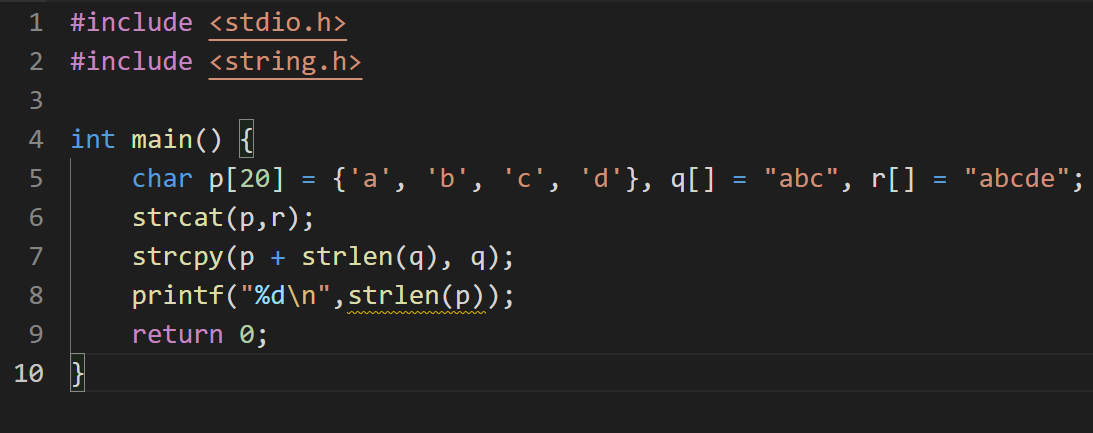## 题目

1. 若有说明int (*p)；则以下 是正确的叙述。

A) p是一个指针数组

B) p是一个指针，它只能指向一个包含3个int类型元素的数组

C) p是一个指针，它可以指向一个一维数组中的任一元素

D) (*p)与*p等价

2. 若有语句int array;以下对下标为i和j的数组元素的引用不正确的是 。

A) array[i][j] B) *(*(array+i)+j) C) *(array[i]+j) D) *(array+i*4+j)

3. 下列不属于C语言关键字的是( ) 。
A) default
B) register
C) enum
D) external

4. C语言源程序的最小单位是( )。

A) 程序行B) 语句C) 函数D) 字符

5. 以下语句或语句组中,能正确进行字符串赋值的是。（ ）

A）char *sp;*sp=\"right!\"; B）char s;s=\"right!\";

C）char s;*s=\"right!\"; D）char *sp=\"right!\";

6. 下列运算符中优先级最高的是（ ）

A、< B、+ C、&& D、==

7. 下面程序段的输出结果是 【】 。

`````` int i=0;
char s1="1234",s2="567";
strcat(s1,s2);
while(s2[i++]!='\0') s2[i]=s1[i];
puts(s2);``````

A) 123 B) 123 567 C) 5234567 D) 523

8. 下面程序段的输出结果是 【】

`````` int a[]={1,2,3,4,5,6,7},*p;
p=a; *(p+3)+=2;
printf ("%d %d\n", *p,*p+3);``````

A) 6 7

B) 1 5

C) 1 6

D) 1 4

9. 下列程序段的输出结果是 【】 。

`````` int i=0;
char s1="1234",s2="567";
strcat(s1,s2);
while(s2[i++]!='\0') s2[i]=s1[i];
puts(s2);``````

A) 123 B) 123 567 C) 5234567 D) 523

10. 设有以下宏定义：#define f(z) z*z，执行赋值语句k=f(4＋4)/f(2＋2)；（k为int型变量）后，k的值是 ___

11. 以下程序的输出结果是( )

``````  main()
{
int x,y,z;
x=y=1;
z=x++,y++,++y;
printf("%d,%d,%d\n",x,y,z);
}``````

A. 2,3,3 B. 2,3,2 C. 2,3,1 D. 2,2,1

12. 设变量均已正确定义，若要通过 scanf("%d%c%d%c",&a1,&c1,&a2,&c2);语句为变量 a1 和 a2 赋数值 10 和 20，为变量 c1 和 c2 赋字符 X 和 Y。以下所示的输入形式中正 确的是（注：□代表空格字符）( )

A. 10□X□20□Y<回车>

B. 10□X20□Y<回车>

C. 10□X<回车>

​ 20□Y<回车>

D. 10X<回车>

​ 20Y<回车>

13. 在 C 语言中，对于以下定义正确的叙述为( )

``````  char x[]="abcdefg";
char y[]={'a','b','c','d','e','f','g'};``````

A. 数组 x 和数组 y 等价 B. 数组 x 和数组 y 的长度相同

C. 数组 x 的长度大于数组 y 的长度 D. 数组 x 的长度小于数组 y 的长度

14. 以下程序的输出结果是( )

``````  main()
{
char str[]="aeiou",*p=str;
printf("%c\n",*p+4));
}``````

A. o B. u C. e D. f

15. 以下程序运行后的输出结果是_____。

``````  main()
{
int a=3,b=4,c=5,t=99;
if(b<a&&a<c) t=a;a=c;c=t;
if(a<c&&b<c) t=b;b=a;a=t;
printf("%d%d%d\n",a,b,c);
}``````
16. 设有定义语句：int a[]={{0},{1},{2}}; 则数组元素 a的值为___

17. 以下程序运行后的输出结果是_____

``````  main(){
printf("%d\n",strlen("IBM\n012\1\\"));
}``````
18. 请选出正确的程序段

A) int *p; scanf("%d"，\&p);

B) int *p; scanf("%d"，p);

C) int k,*p=&k; scanf("%d"，p);

D) int k,p;\p=&k; scanf("%d"，p);

19. 若有说明 ;int *p,m=5,n; 以下正确的程序段的是____.

A.p=&n;scanf("%d",&p)

B.p=&n;scanf("%d",*p);

C.scanf("%d",&n);*p=n;

D.p=&n;*p=m;

20. 有以下定义：int a；long b；double x，y；则以下选项中正确的表达式是（　　）。

A．a%(int)(x-y)
B．a=x!=y；
C．(a*y)%b
D．y=x+y=x

21. 程序运行后的结果是( )1. B

2. D

3. D

4. B

5. D

6. B

7. C

8. D

9. C

10. 28

11. A

12. D

13. C

14. C

15. 4599

16. 0

17. 9

18. C

19. D

20. B

21. 6